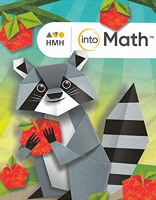1 Unit 1 Numbers to 20 and Data Module 1 - Fluency for Addition and Subtraction Within 20 Lesson 1:  Use Doubles Facts to Add Also consider: Lesson 1:  Use Doubles Facts to Add Also consider: Lesson 2:  Develop Fluency with Addition Using Mental Strategies and Properties 2. Also consider: Lesson 2:  Develop Fluency with Addition Using Mental Strategies and Properties 2. Also consider: Lesson 3:  Relate Addition and Subtraction Also consider: Lesson 3:  Relate Addition and Subtraction Also consider: Lesson 4:  Develop Fluency with Subtraction Using Mental Strategies Also consider: Lesson 4:  Develop Fluency with Subtraction Using Mental Strategies Also consider: Lesson 5:  Use the Make a Ten Strategy to Add Also consider: Lesson 5:  Use the Make a Ten Strategy to Add Also consider: Lesson 6:  Use a Tens Fact to Subtract Lesson 6:  Use a Tens Fact to Subtract Lesson 7:  Add 3 Numbers Using Mental Stategies and Properties Lesson 7:  Add 3 Numbers Using Mental Stategies and Properties Module 2 - Equal Groups Lesson 1:  Identify Even and Odd Numbers Also consider: Lesson 1:  Identify Even and Odd Numbers Also consider: Lesson 2:  Write Equations to Represent Even Numbers Lesson 2:  Write Equations to Represent Even Numbers Lesson 3:  Represent Equal Groups Lesson 3:  Represent Equal Groups Lesson 4:  Add to Find the Total Number of Objects in Arrays Lesson 4:  Add to Find the Total Number of Objects in Arrays Lesson 5:  Practice with Arrays Also consider: Lesson 5:  Practice with Arrays Also consider: Module 3 - Data Lesson 1:  Collect and Record Data Lesson 1:  Collect and Record Data Lesson 2:  Interpret Picture Graphs Lesson 2:  Interpret Picture Graphs Lesson 3:  Draw Picture Graphs to Represent Data Lesson 3:  Draw Picture Graphs to Represent Data Lesson 4:  Interpret Bar Graphs Lesson 4:  Interpret Bar Graphs Lesson 5:  Draw Bar Graphs to Represent Data Also consider: Lesson 5:  Draw Bar Graphs to Represent Data Also consider:
 2 Unit 2 Place Value Module 4 - Understand Place Value Lesson 1:  Group Tens as Hundreds Lesson 1:  Group Tens as Hundreds Lesson 2:  Understand Three-Digit Numbers Lesson 2:  Understand Three-Digit Numbers Lesson 3:  Represent Three-Digit Numbers Lesson 3:  Represent Three-Digit Numbers Lesson 4:  Represent Numbers with Hundreds, Tens, and Ones Lesson 4:  Represent Numbers with Hundreds, Tens, and Ones Lesson 5:  Place Value to 1,000 Lesson 5:  Place Value to 1,000 Module 5 - Read, Write, and Show Numbers to 1,000 Lesson 1:  Use Expanded Form Lesson 1:  Use Expanded Form Lesson 2:  Use Number Names Lesson 2:  Use Number Names Lesson 3:  Different Ways to Write Numbers Lesson 3:  Different Ways to Write Numbers Lesson 4:  Different Ways to Show Numbers Lesson 4:  Different Ways to Show Numbers Lesson 5:  Read, Write, and Show Numbers Lesson 5:  Read, Write, and Show Numbers Module 6 - Use Place Value Lesson 1:  Count Within 1,000 Lesson 1:  Count Within 1,000 Lesson 2:  Add and Subtract 10 or 100 Lesson 2:  Add and Subtract 10 or 100 Lesson 3:  Identify and Extend Number Patterns Also consider: Lesson 3:  Identify and Extend Number Patterns Also consider: Lesson 4:  Compare Three-Digit Numbers Lesson 4:  Compare Three-Digit Numbers Lesson 5:  Use Symbols to Compare Numbers Also consider: Lesson 5:  Use Symbols to Compare Numbers Also consider:
 3 Unit 3 Money and Time Module 7 - Coins Lesson 1:  Relate Place Value to Coins Lesson 1:  Relate Place Value to Coins Lesson 2:  Identify and Find the Value of Coins Lesson 2:  Identify and Find the Value of Coins Lesson 3:  Compute the value of Coin Combinations Lesson 3:  Compute the value of Coin Combinations Lesson 4:  Show Amounts in Different Ways Also consider: Lesson 4:  Show Amounts in Different Ways Also consider: Module 8 - Dollar Amounts Lesson 1:  Relate the Value of Coins to One Dollar Lesson 1:  Relate the Value of Coins to One Dollar Lesson 2:  Compute the Value of Dollar Combinations Lesson 2:  Compute the Value of Dollar Combinations Lesson 3:  Solve Problems Involving Money Also consider: Lesson 3:  Solve Problems Involving Money Also consider: Module 9 - Time Lesson 1:  Tell and Write Time to 5 Minutes Also consider: Lesson 1:  Tell and Write Time to 5 Minutes Also consider: Lesson 2:  Different Ways to Tell and Write Time Lesson 2:  Different Ways to Tell and Write Time Lesson 3:  Practice Telling and Writing Time Lesson 3:  Practice Telling and Writing Time Lesson 4:  Tell and Write Time with A.M. and P.M. Lesson 4:  Tell and Write Time with A.M. and P.M.